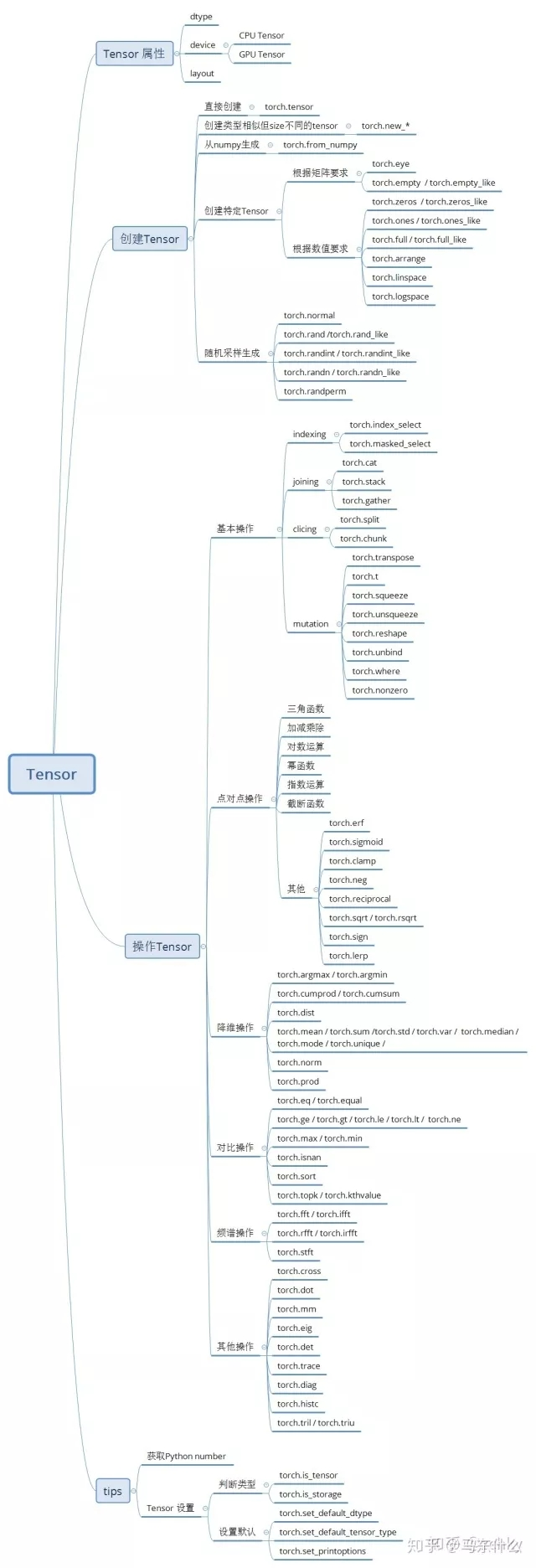# pytorch入门总结指南（1）

2019-12-16 10:09| 发布者: 炼数成金_小数| 查看: 42831| 评论: 0|来自: AI开发者

 本来觉得学个tf和keras就够了，但是用了torch之后觉得真的这个框架太好用了，非常灵活可以很方便的和python语言混编，torch基本可以和cupy并列称为gpu版的numpy，文本部分有torchtext和allenlp，调包有sktorch非常简单的融入到sklearn的生态中，再高层的封装有fast.ai，简直太香了。考虑到网上的入门教程多且杂，还是打算自己好好整理一下，系统性的学习一下pytroch。https://github.com/bharathgs/Awesome-pytorch-listpytorch的各类详细资源列表。总结内容包括了，github上的各类高星tutorial（其实内容上基本差不多大同小异的），pytorch中文手册，《deep learning with pytorch》《深度学习框架pytorch快速开发与实战》，《深度学习入门之torch》以及官方文档，说老实话，大部分教程或者书讲的都差不多，还是官网提供的信息量最多较大。关于torch的背景介绍就算了，网上一大堆，主要讲一下torch的主要功能：1、和tensorflow、mxnet一样，用于构建各类dl模型；2、对我来说torch较大的优势之一，当gpu加速版的numpy来用，类似于cupy、cudf这类，cupy目前windows版本支持，而gpu版本的cudf暂时只能在Linux下跑，至于rapidsai的cuml，目前功能还是不太完善很多算法没有。3、也是很重要的一点，tf的原始接口写nn虽然灵活但是麻烦，keras虽然简单但是要做灵活的改变却显得麻烦，pytorch处于比较折中的位置，不像keras那样上手就来（不过torch现在也支持sequential构建nn的功能所以实际上也已经非常简单耐用了），不过引入skorch之后方便得多，很灵活的就能和现有的代码结合起来。而且可以非常灵活简单的参与到nn的训练过程中来。显然，关于torch的使用，一开始就得介绍tensor（张量）的概念，张量很简单。一个点叫0阶张量，一维数据（向量）是一阶张量，二维数组（矩阵）是二阶张量，三维数组就叫三阶张量，张量是矢量概念的一种推广。就api来说，torch.tensor和numpy的接口非常类似，事实上，torch也提供了numpy和tensor之间转换的接口。zcyanqiu：pytorch入坑一 | Tensor及其基本操作https://zhuanlan.zhihu.com/p/36233589这个思维导图是真的nice，可以看到torch.实现了基本上所有常用的numpy的处理方法。和cupy还真是。。。相似呢。下面我们用torch和cupy分别重写一下之前用numpy写的逻辑回归，这一章就结束。下面是纯numpy撸的简单版逻辑回归的代码首先导入样本数据：from sklearn.datasets import load_breast_cancerX=load_breast_cancer().datay=load_breast_cancer().target然后是纯numpy撸的简单版逻辑回归：import numpy as npdef sigmoid(inX):#定义sigmoid函数    return 1.0/(1+np.exp(-inX))def gradAscent(dataMatIn, classLabels):    #dataMatrix = np.mat(dataMatIn)             #    #labelMat = np.mat(classLabels).transpose() #这两行不用太在意，和算法没关系就是格式转换而已#labelMat是0-1标签值，dataMatrix是特征矩阵    dataMatrix=dataMatIn    labelMat=classLabels.reshape(-1,1)    m,n = np.shape(dataMatrix)    alpha = 0.001 #学习率，用于调整梯度下降的步幅    maxCycles = 500 #较大迭代次数，因为这里直接使用的是批梯度下降法（BGD），所以这里的迭代次数#就是使用全部数据计算全部样本的损失函数的值的并进行统一的梯度更新 这个过程重复多少次    weights = np.ones((n,1)) #权重和偏置统一放在一起变成一个大权重，这样计算方便的多#这里使用的是全1初始化，lr对于初始化并不敏感    for k in range(maxCycles):        h = sigmoid(np.dot(dataMatrix,weights))     #        error =  h.reshape(-1,1)-labelMat              #这句就是根据上面求的梯度更新量的那个公式得到的        weights = weights - alpha * np.dot(dataMatrix.T, error) #因为是梯度下降所以每次都是减去#相应的梯度更新量，这里就是根据前面求解出来的公式得到梯度更新量 的表达式    return weights然后是cupy撸的：import cupy as npdef sigmoid(inX):#定义sigmoid函数    return 1.0/(1+np.exp(-inX))def gradAscent(dataMatIn, classLabels):    #dataMatrix = np.mat(dataMatIn)             #    #labelMat = np.mat(classLabels).transpose() #这两行不用太在意，和算法没关系就是格式转换而已#labelMat是0-1标签值，dataMatrix是特征矩阵    dataMatrix=dataMatIn    labelMat=classLabels.reshape(-1,1)    m,n = np.shape(dataMatrix)    alpha = 0.001 #学习率，用于调整梯度下降的步幅    maxCycles = 500 #较大迭代次数，因为这里直接使用的是批梯度下降法（BGD），所以这里的迭代次数#就是使用全部数据计算全部样本的损失函数的值的并进行统一的梯度更新 这个过程重复多少次    weights = np.ones((n,1)) #权重和偏置统一放在一起变成一个大权重，这样计算方便的多#这里使用的是全1初始化，lr对于初始化并不敏感    for k in range(maxCycles):        h = sigmoid(np.dot(dataMatrix,weights))     #        error =  h.reshape(-1,1)-labelMat              #这句就是根据上面求的梯度更新量的那个公式得到的        weights = weights - alpha * np.dot(dataMatrix.T, error) #因为是梯度下降所以每次都是减去#相应的梯度更新量，这里就是根据前面求解出来的公式得到梯度更新量 的表达式    return weights最后用pytorch撸的：import torch as npdef sigmoid(inX):#定义sigmoid函数    return 1.0/(1+torch.exp(-inX))def gradAscent(dataMatIn, classLabels):    #dataMatrix = np.mat(dataMatIn)             #    #labelMat = np.mat(classLabels).transpose() #这两行不用太在意，和算法没关系就是格式转换而已#labelMat是0-1标签值，dataMatrix是特征矩阵    dataMatrix=dataMatIn    labelMat=classLabels.reshape(-1,1)    m,n = dataMatrix.shape    alpha = 0.001 #学习率，用于调整梯度下降的步幅    maxCycles = 500 #较大迭代次数，因为这里直接使用的是批梯度下降法（BGD），所以这里的迭代次数#就是使用全部数据计算全部样本的损失函数的值的并进行统一的梯度更新 这个过程重复多少次    weights = np.ones((n,1),dtype=float) #权重和偏置统一放在一起变成一个大权重，这样计算方便的多#这里使用的是全1初始化，lr对于初始化并不敏感    for k in range(maxCycles):        h = sigmoid(np.mm(dataMatrix,weights))     #        error =  h.reshape(-1,1)-labelMat              #这句就是根据上面求的梯度更新量的那个公式得到的        weights = weights - alpha * np.mm(dataMatrix.T, error) #因为是梯度下降所以每次都是减去#相应的梯度更新量，这里就是根据前面求解出来的公式得到梯度更新量 的表达式    return weights整体上差别不大，就是pytorch中dot只能针对一维数组，也就是shape为(m,)这样的矩阵，而如果是多维数组则需要使用mm，需要注意的是无论是numpy，cupy还是pytorch，矩阵的直接 “*”都是哈达玛积，也就是各位相乘不求和，而dot或者mm才是正常的矩阵相乘，也就是我们初高中熟悉的“正经”的矩阵乘法，需要注意。可以看到，torch和numpy之间的差异性很小，如果使用cuda，直接把数据.cuda()到gpu上，那么接下去的运算就都会自动在gpu上运行了，贼方便。最后用torch的nn撸一个简单快速的：class LR(nn.Module):    def __init__(self):        super(LR,self).__init__()        self.fc=nn.Linear(30,2) #        self.activation=torch.sigmoid    def forward(self,x):        out=self.fc(x)        out=self.activation(out)        return outnet=LR()criterion=nn.CrossEntropyLoss() # 使用CrossEntropyLoss损失optm=torch.optim.Adam(net.parameters(),lr=0.1) # Adam优化epochs=1000 # 训练1000次for i in range(epochs):    # 指定模型为训练模式，计算梯度    net.train()    # 输入值都需要转化成torch的Tensor    x=torch.from_numpy(X).float()    y=torch.from_numpy(Y).long()    y_hat=net(x)    loss=criterion(y_hat,y) # 计算损失    optm.zero_grad() # 前一步的损失清零    loss.backward() # 反向传播    optm.step() # 优化net.eval() #结束训练，固定权重 偏置，进入测试（预测）模式net(torch.from_numpy(X).float())可以看到pytorch的forward的过程和keras的function api形式一模一样，而torch.nn.sequential也和keras的sequntial模式基本相同，从keras转torch难度很低的。这么写挺麻烦的，感谢开源的skorch，让这一切变得简单无比：X=load_breast_cancer().dataY=load_breast_cancer().targetX=X.astype('float32')Y=Y.astype('int64')class LR(nn.Module):    def __init__(self):        super(LR,self).__init__()        self.fc=nn.Linear(30,2) #        self.activation=torch.sigmoid    def forward(self,x):        out=self.fc(x)        out=self.activation(out)        return outfrom skorch import NeuralNetClassifiernet = NeuralNetClassifier(    LR,    max_epochs=1000,    criterion=nn.CrossEntropyLoss,    train_split=None,    optimizer=torch.optim.Adam,    optimizer__lr=0.1,    warm_start=True,    verbose=False,    batch_size=256    # Shuffle training data on each epoch)net.fit(X, Y)y_proba = net.predict_proba(X)需要注意的是，连续值必须转化为float32格式，离散值必须转化为int64，否则会报错。。。。无语底层代码不难，有空也可以自己改改。这样包装之后的net就可以和gridsearch，scikit-optimize等一切sklearn-api的其它库之间形成良好的交互了。skorch.classifier - skorch 0.7.0 documentationhttps://skorch.readthedocs.io/en/latest/classifier.html如果需要原始网络结构的属性，直接访问module这个属性就可以了。声明：文章收集于网络，版权归原作者所有，为传播信息而发，如有侵权，请联系小编删除，谢谢！欢迎加入本站公开兴趣群商业智能与数据分析群兴趣范围包括：各种让数据产生价值的办法，实际应用案例分享与讨论，分析工具，ETL工具，数据仓库，数据挖掘工具，报表系统等全方位知识QQ群：81035754

## 热门频道

• 大数据
• 商业智能
• 量化投资
• 科学探索
• 创业GMT+8, 2020-3-30 14:29 , Processed in 0.164857 second(s), 24 queries .